# KR101426877B1 - Rotation angle detection device, rotary machine, and rotation angle detection method - Google Patents

Rotation angle detection device, rotary machine, and rotation angle detection method Download PDF

## Info

Publication number
KR101426877B1
KR101426877B1 KR1020107015873A KR20107015873A KR101426877B1 KR 101426877 B1 KR101426877 B1 KR 101426877B1 KR 1020107015873 A KR1020107015873 A KR 1020107015873A KR 20107015873 A KR20107015873 A KR 20107015873A KR 101426877 B1 KR101426877 B1 KR 101426877B1
Authority
KR
South Korea
Prior art keywords
sensor
magnetic flux
rotation angle
magnet
flux density
Prior art date
Application number
KR1020107015873A
Other languages
Korean (ko)
Other versions
KR20100126273A (en
Inventor
마사히로 미타
교헤이 아이무타
오사무 시모에
리히토 가가와
Original Assignee
히타치 긴조쿠 가부시키가이샤
Priority date (The priority date is an assumption and is not a legal conclusion. Google has not performed a legal analysis and makes no representation as to the accuracy of the date listed.)
Filing date
Publication date
Priority to JPJP-P-2008-027636 priority Critical
Priority to JP2008027636 priority
Priority to JPJP-P-2008-116246 priority
Priority to JP2008116246 priority
Application filed by 히타치 긴조쿠 가부시키가이샤 filed Critical 히타치 긴조쿠 가부시키가이샤
Priority to PCT/JP2009/051768 priority patent/WO2009099054A1/en
Publication of KR20100126273A publication Critical patent/KR20100126273A/en
Application granted granted Critical
Publication of KR101426877B1 publication Critical patent/KR101426877B1/en

• 238000001514 detection method Methods 0.000 title claims description 6
• 230000004907 flux Effects 0.000 claims abstract description 136
• 239000010410 layers Substances 0.000 claims description 57
• 230000005415 magnetization Effects 0.000 claims description 54
• 230000014509 gene expression Effects 0.000 claims description 10
• 238000009434 installation Methods 0.000 claims description 5
• 230000000737 periodic Effects 0.000 claims description 3
• 240000001016 Solanum tuberosum Species 0.000 description 26
• 235000002595 Solanum tuberosum Nutrition 0.000 description 26
• 230000000052 comparative effects Effects 0.000 description 25
• 238000010586 diagrams Methods 0.000 description 15
• 238000004364 calculation methods Methods 0.000 description 10
• 230000000694 effects Effects 0.000 description 8
• 235000020127 ayran Nutrition 0.000 description 6
• 238000000034 methods Methods 0.000 description 6
• 238000006243 chemical reactions Methods 0.000 description 5
• 229910001172 neodymium magnets Inorganic materials 0.000 description 5
• 230000002093 peripheral Effects 0.000 description 5
• 230000003321 amplification Effects 0.000 description 3
• 238000000465 moulding Methods 0.000 description 3
• 238000003199 nucleic acid amplification method Methods 0.000 description 3
• 230000000875 corresponding Effects 0.000 description 2
• 239000000696 magnetic materials Substances 0.000 description 2
• 239000000463 materials Substances 0.000 description 2
• 239000000758 substrates Substances 0.000 description 2
• 230000001629 suppression Effects 0.000 description 2
• 241001081830 Degeneriaceae Species 0.000 description 1
• 102200082816 HBB S45C Human genes 0.000 description 1
• 229910001030 Iron–nickel alloy Inorganic materials 0.000 description 1
• 241000232219 Platanista Species 0.000 description 1
• 230000004323 axial length Effects 0.000 description 1
• 239000011230 binding agents Substances 0.000 description 1
• 239000011651 chromium Substances 0.000 description 1
• 239000011248 coating agents Substances 0.000 description 1
• 238000000576 coating method Methods 0.000 description 1
• 239000010949 copper Substances 0.000 description 1
• 238000006073 displacement reactions Methods 0.000 description 1
• 238000009413 insulation Methods 0.000 description 1
• 239000011514 iron Substances 0.000 description 1
• 229910052742 iron Inorganic materials 0.000 description 1
• 238000000691 measurement method Methods 0.000 description 1
• 238000005259 measurements Methods 0.000 description 1
• 230000005405 multipole Effects 0.000 description 1
• 239000000843 powders Substances 0.000 description 1
• 239000011347 resins Substances 0.000 description 1
• 229920005989 resins Polymers 0.000 description 1
• 238000007493 shaping process Methods 0.000 description 1
• 238000004088 simulation Methods 0.000 description 1
• 239000007787 solids Substances 0.000 description 1

## Images

••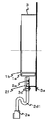•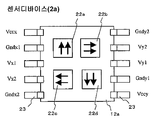•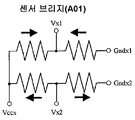•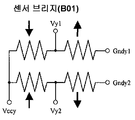•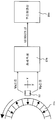•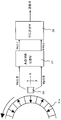•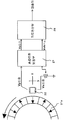•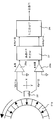••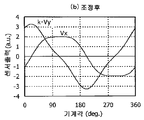•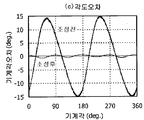••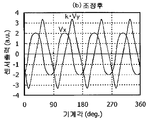•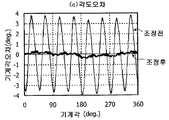•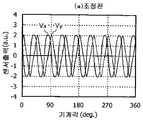•••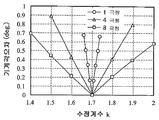••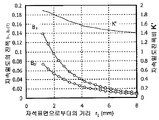•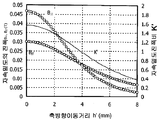•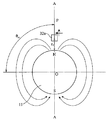•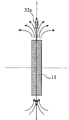•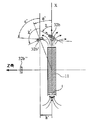••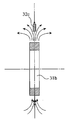••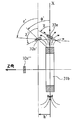•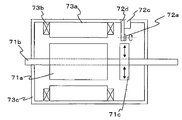•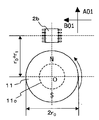•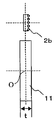•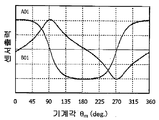•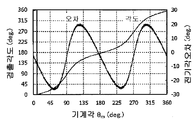••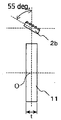•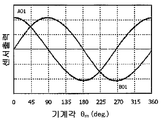•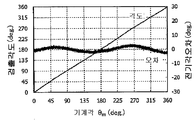•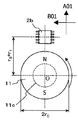••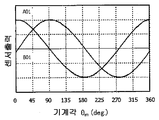•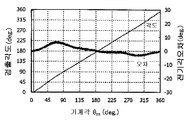•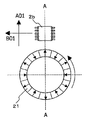•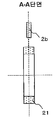•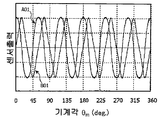•••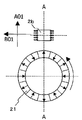•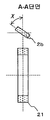•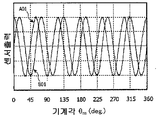•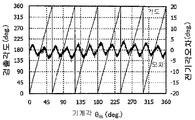•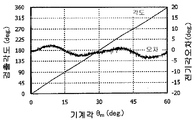••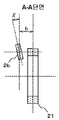•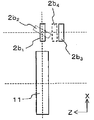•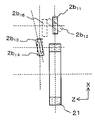•••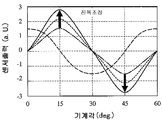•## Classifications

• GPHYSICS
• G01MEASURING; TESTING
• G01DMEASURING NOT SPECIALLY ADAPTED FOR A SPECIFIC VARIABLE; ARRANGEMENTS FOR MEASURING TWO OR MORE VARIABLES NOT COVERED IN A SINGLE OTHER SUBCLASS; TARIFF METERING APPARATUS; MEASURING OR TESTING NOT OTHERWISE PROVIDED FOR
• G01D5/00Mechanical means for transferring the output of a sensing member; Means for converting the output of a sensing member to another variable where the form or nature of the sensing member does not constrain the means for converting; Transducers not specially adapted for a specific variable
• G01D5/12Mechanical means for transferring the output of a sensing member; Means for converting the output of a sensing member to another variable where the form or nature of the sensing member does not constrain the means for converting; Transducers not specially adapted for a specific variable using electric or magnetic means
• G01D5/14Mechanical means for transferring the output of a sensing member; Means for converting the output of a sensing member to another variable where the form or nature of the sensing member does not constrain the means for converting; Transducers not specially adapted for a specific variable using electric or magnetic means influencing the magnitude of a current or voltage
• G01D5/142Mechanical means for transferring the output of a sensing member; Means for converting the output of a sensing member to another variable where the form or nature of the sensing member does not constrain the means for converting; Transducers not specially adapted for a specific variable using electric or magnetic means influencing the magnitude of a current or voltage using Hall-effect devices
• G01D5/145Mechanical means for transferring the output of a sensing member; Means for converting the output of a sensing member to another variable where the form or nature of the sensing member does not constrain the means for converting; Transducers not specially adapted for a specific variable using electric or magnetic means influencing the magnitude of a current or voltage using Hall-effect devices influenced by the relative movement between the Hall device and magnetic fields
• GPHYSICS
• G01MEASURING; TESTING
• G01DMEASURING NOT SPECIALLY ADAPTED FOR A SPECIFIC VARIABLE; ARRANGEMENTS FOR MEASURING TWO OR MORE VARIABLES NOT COVERED IN A SINGLE OTHER SUBCLASS; TARIFF METERING APPARATUS; MEASURING OR TESTING NOT OTHERWISE PROVIDED FOR
• G01D5/00Mechanical means for transferring the output of a sensing member; Means for converting the output of a sensing member to another variable where the form or nature of the sensing member does not constrain the means for converting; Transducers not specially adapted for a specific variable
• G01D5/12Mechanical means for transferring the output of a sensing member; Means for converting the output of a sensing member to another variable where the form or nature of the sensing member does not constrain the means for converting; Transducers not specially adapted for a specific variable using electric or magnetic means
• G01D5/244Mechanical means for transferring the output of a sensing member; Means for converting the output of a sensing member to another variable where the form or nature of the sensing member does not constrain the means for converting; Transducers not specially adapted for a specific variable using electric or magnetic means influencing characteristics of pulses or pulse trains; generating pulses or pulse trains
• G01D5/24428Error prevention
• G01D5/24433Error prevention by mechanical means
• G01D5/24438Special design of the sensing element or scale
• GPHYSICS
• G01MEASURING; TESTING
• G01DMEASURING NOT SPECIALLY ADAPTED FOR A SPECIFIC VARIABLE; ARRANGEMENTS FOR MEASURING TWO OR MORE VARIABLES NOT COVERED IN A SINGLE OTHER SUBCLASS; TARIFF METERING APPARATUS; MEASURING OR TESTING NOT OTHERWISE PROVIDED FOR
• G01D5/00Mechanical means for transferring the output of a sensing member; Means for converting the output of a sensing member to another variable where the form or nature of the sensing member does not constrain the means for converting; Transducers not specially adapted for a specific variable
• G01D5/12Mechanical means for transferring the output of a sensing member; Means for converting the output of a sensing member to another variable where the form or nature of the sensing member does not constrain the means for converting; Transducers not specially adapted for a specific variable using electric or magnetic means
• G01D5/244Mechanical means for transferring the output of a sensing member; Means for converting the output of a sensing member to another variable where the form or nature of the sensing member does not constrain the means for converting; Transducers not specially adapted for a specific variable using electric or magnetic means influencing characteristics of pulses or pulse trains; generating pulses or pulse trains
• G01D5/24471Error correction
• G01D5/2448Correction of gain, threshold, offset or phase control

## Abstract

The present invention relates to a rotation angle detecting device for detecting a rotation angle by using a magnet rotor (N is a natural number) having a 2N pole magnet and a sensor device for detecting the direction of a magnetic flux from the magnet rotor , At least one of the two output voltage values in the radial direction and the rotational direction obtained by the sensor device is multiplied by a correction coefficient and the rotation angle is calculated from the two corrected output voltage values, The rotation angle detection device being characterized in that:

## Description

TECHNICAL FIELD  The present invention relates to a rotation angle detection device, a rotation angle detection method, and a rotation angle detection method.

BACKGROUND OF THE INVENTION 1. Field of the Invention The present invention relates to a rotation angle detecting device for detecting a rotation angle of a rotation shaft and the like, a rotary device using the same, and a rotation angle detecting method thereof.

When a cylindrical magnet having a peripheral surface magnetized with a plurality of poles is rotated around the axis of the cylinder, a magnetic flux in a radial direction of the cylindrical magnet at a position (r 1 ) Flux density) and the magnetic flux density in the circumferential direction change in a substantially sinusoidal waveform with respect to the rotation angle, respectively. At this time, the amplitude of the magnetic flux density in the radial direction becomes 1 to 2 times larger than the amplitude of the magnetic flux density in the rotating direction.

A sensor device (a magnetic sensor using a plurality of spin valve type giant magnetoresistive elements) is disposed at a position apart from the distance r 1 and the magnetic flux density in the radial direction and the magnetic flux density in the rotational direction are measured, Can be configured.

If the amplitude of the magnetic flux density in the radial direction is different from the amplitude of the magnetic flux density in the rotational direction because the sensor device detects the direction of the magnetic flux instead of the intensity of the magnetic field, the sensor device having the magnetostrictive axis in the radial direction It is possible to obtain a first output voltage V x having a trapezoidal waveform and a second output voltage V y having a triangular waveform on the sensor device having the potato axis in the rotation direction. Here, the first and second output voltages can be obtained at the same time by using a sensor device in which two orthogonal potato axes are concentrated on one substrate.

Further, if the magnetic flux density is within a certain range, the amplitude of the output voltage becomes substantially constant irrespective of the maximum value of the magnetic flux density in the radial direction and the rotational direction. That is, the first and second output voltages become V x = cos θ mag and V y = sin θ mag , respectively, with respect to the rotation angle θ mag of the magnet as will be described later, The resulting waveform includes harmonics, so that the waveforms are trapezoidal and triangular, respectively). As described above, the first and second output voltages have different waveforms, and an output voltage having substantially the same amplitude can be obtained despite the difference in amplitude of the magnetic flux density, resulting in a measurement error (angular error) of the rotation angle.

Japanese Patent Application Laid-Open No. 2006-023179 (Patent Document 1) discloses a magnetic sensor comprising a magnetic member and a plurality of pairs of vector detection type magnetoresistance effect elements facing the magnetic pole arrangement surface of the magnetic member, The vector detection type magnetoresistance effect element is arranged so that the magnetization directions of the pinned layer magnetization directions of the pair of vector detection type magnetoresistance effect elements are substantially parallel to the external magnetic field by the magnetic member, And all of the vector detection type magnetoresistive elements are disposed at the same position with respect to the magnetic pole array direction of the magnetic member. In the magnetic position detection apparatus disclosed in Patent Document 1, And a vector detection type magnetoresistive element are relatively moved to obtain a two-phase sinusoidal output whose phase is shifted by 90 degrees.

However, as described in Japanese Patent Laid-Open Publication No. 2006-023179 (Patent Document 1), it is possible to obtain a two-phase sinusoidal output whose phase is shifted by 90 degrees because the magnetic flux density amplitude ratio in the moving direction and in the direction perpendicular to the magnetic member is approximately 1 (in the case where the lateral dimension of the magnetic pole is long relative to the magnetizing pitch of the magnetic member), and actually the magnetic flux density amplitude is different, the output voltage from the magnetic field detector is a trapezoidal waveform And a triangular wave waveform. As a result, an error occurs in the position detection, and accurate measurement can not be performed.

Japanese Patent Application Laid-Open No. 2006-194861 (Patent Document 2) discloses a technique for detecting a rotation angle by using a magneto-resistive effect element, such as a phase error and a nonuniformity error between signals included in the output from the magneto- Is reduced by various waveform shaping. However, a method for reducing the error caused by nonuniformity (difference in amplitude ratio of the external magnetic field, etc.) principally included in the magnetic field generated by the rotation of the parallel magnetic field is not disclosed.

Since the amplitude of the magnetic flux density in the radial direction is larger than the amplitude of the magnetic flux density in the rotational direction, as disclosed in Japanese Patent Laid-Open Nos. 2006-023179 and 2006-194861 (Patent Literature 2) The waveforms of the output voltages in the direction and the rotational direction are different from each other, and the resulting angular error is not described, and there is no such indication. In the technique described in these documents, in order to make the amplitude of the magnetic flux density in the moving direction equal to the amplitude of the magnetic flux density in the vertical direction from the magnetic member, it is necessary to make the lateral dimension of the magnetic pole to be about 100 times, for example, . However, it is not realistic to install such a large magnetic member for detecting the rotation angle or the moving distance.

As described above, when a sensor device capable of detecting the direction of the magnetic flux is used, in order to obtain the electric angle (electric angle) and the rotation angle of the magnet rotor without calculating the two output voltages as they are, There has been a problem in that it can only be performed by a rotation angle detecting device having a large angular error.

Accordingly, it is an object of the present invention to provide a rotation angle detecting device capable of detecting a rotation angle with high precision, and a rotating machine having the same.

As a result of intensive studies in view of the above object, the present inventors have found that, in a rotational angle detecting apparatus using a sensor device for detecting the direction of magnetic flux, two output voltages in the radial direction and rotational direction (Or divided), and the ratio of the maximum value of the two output voltages (or the output voltage waveform) is calculated in the radial direction and the rotational direction at the position where the sensor device is disposed Direction of the magnetic flux density of the magnetic flux density of the magnetic flux density of the magnetic flux density of the magnetic flux density of the magnetic flux density in the direction of the magnetic flux density.

27, the amplitude of at least one of the obtained output voltage waveforms is enlarged and the ratio of the maximum value of the two output voltage waveforms is set to the maximum value of the corresponding two magnetic flux densities And then the rotation angle or the mechanical angle is obtained. As the adjusting means, either of a method of enlarging only one side, a method of reducing only one side, or a method of applying a predetermined coefficient to both sides may be used. As a result, the ratio of the maximum value of the two output voltage waveforms K '. Hereinafter, the multiplication of the correction coefficient on one side will be mainly described, but the present invention is not limited to this, and the other may be modified or modified by applying a predetermined coefficient to both sides.

That is, in the rotation angle detecting device of the present invention,

1. A rotation angle detecting device for detecting a rotation angle by using a magnet rotor (N is a natural number) having a 2N pole magnet and a sensor device for detecting a direction of a magnetic flux from the magnet rotor,

Characterized in that at least one of the two output voltage values in the radial direction and the rotational direction obtained by the sensor device is multiplied by a correction coefficient and the rotation angle is calculated from the two corrected output voltage values, do.

Another rotation angle detecting device of the present invention includes:

1. A rotation angle detecting device comprising a magnet rotor (N is a natural number) having a 2N pole magnet and a sensor device for detecting the direction of a magnetic flux from the magnet rotor,

The sensor device includes a plurality of spin valve type giant magnetoresistive elements (a magnetoresistive element having a pinned layer and a free layer fixed in a fixed layer magnetization direction and a free layer magnetization direction rotating in the direction of the magnetic flux) And a sensor bridge A01 and a sensor bridge B01 in which the fixed layer magnetization directions are orthogonal to each other,

The sensor bridge A01 and the sensor bridge B01 are bridge circuits of the spin valve type giant magnetoresistive element,

In the bridge circuit, magnetization directions of fixed layer magnetization directions of the spin valve type giant magnetoresistive elements on the electrically adjacent sides are antiparallel,

An output voltage according to an angle formed by the fixed layer magnetization direction and the free layer magnetization direction is obtained by applying a voltage to each of the sensor bridge A01 and the sensor bridge B01,

And an angle signal is obtained based on two outputs obtained by multiplying at least one of the output voltages by a correction coefficient.

Wherein the sensor device comprises a plurality of spin valve type giant magnetoresistive elements having a pinned layer and a free layer and fixed in two directions in which the pinned layer magnetization directions are orthogonal to each other and in which the free layer magnetization direction rotates along the direction of the magnetic flux Element,

The correction factor is preferably a value reflecting the ratio between the maximum value of the magnetic flux density in the radial direction and the maximum value of the magnetic flux density in the rotational direction at the position where the sensor device is installed.

In a position to install the sensor device, when the ratio of the amplitude B // of the amplitude B magnetic flux density in the rotational direction of the magnetic flux density in the radial direction in B ⊥ / B // = K ' , the radius after the modified The ratio K of the peak value of the output voltage value in the direction of rotation to the peak value of the output voltage value in the rotation direction is preferably in the range of K = K '± 0.3N.

In another rotation angle detecting device of the present invention,

1. A rotation angle detecting device for detecting a rotation angle by using a magnet rotor (N is a natural number) having a 2N pole magnet and a sensor device for detecting a direction of a magnetic flux from the magnet rotor,

At least one of amplitude values of two output voltage values in the radial direction and the rotational direction obtained by the sensor device is multiplied by a correction coefficient and the rotation angle is calculated from the two modified output waveforms, .

Wherein the sensor device comprises a plurality of spin valve type giant magnetoresistive elements having a pinned layer and a free layer and fixed in two directions in which the pinned layer magnetization directions are orthogonal to each other and in which the free layer magnetization direction rotates along the direction of the magnetic flux Element,

It is preferable that the correction factor is a value reflecting the ratio of the respective maximum amplitudes when the waveform of the magnetic flux density in the two directions of the radial direction and the rotational direction is taken at the position where the sensor device is installed.

In a position to install the sensor device, when the ratio of the amplitude B // of the amplitude B magnetic flux density in the rotational direction of the magnetic flux density in the radial direction in B ⊥ / B // = K ' , the radius after the modified The ratio K between the peak value of the output waveform in the direction of rotation and the peak value of the output waveform in the rotation direction is preferably a value in the range of K = K '± 0.3N.

In another rotation angle detecting device of the present invention,

1. A rotation angle detecting device comprising a magnet rotor (N is a natural number) having a 2N pole magnet and a sensor device for detecting the direction of a magnetic flux from the magnet rotor,

The sensor device includes a plurality of spin valve type giant magnetoresistive elements (a magnetoresistive element having a pinned layer and a free layer fixed in a fixed layer magnetization direction and a free layer magnetization direction rotating in the direction of the magnetic flux) And a sensor bridge A01 and a sensor bridge B01 in which the fixed layer magnetization directions are orthogonal to each other,

The sensor bridge A01 and the sensor bridge B01 are bridge circuits of the spin valve type giant magnetoresistive element,

In the bridge circuit, magnetization directions of fixed layer magnetization directions of the spin valve type giant magnetoresistive elements on the electrically adjacent sides are antiparallel,

(V x , V y ) according to an angle formed by the fixed layer magnetization direction and the free layer magnetization direction by applying a voltage to each of the sensor bridge A01 and the sensor bridge B01,

And an angle signal is obtained by multiplying at least one of the output voltages by a correction coefficient.

[However, V x is the output voltage of the sensor bridge (A01) towards the fixing layer the magnetization direction in a radial direction of the magnet rotor,

V y is the output voltage of the sensor bridge B 01 toward the fixed layer magnetization direction in the rotating direction of the magnet rotor,

The correction coefficient is a value obtained by multiplying each of V x and V y by a 1 , a 3 , and a 1 in the following equations (1-1) and (1-2) in which one electrical period is fourier- b 1, and b 3 .

It is preferable to use k as the correction coefficient.

(Where N is the maximum number of the magnet rotors and k 'is the number of revolutions per unit time in the above formulas (1-1) and (1-2)), By the numerical values of a 1 , a 3 , b 1 and b 3 , the equation (2):

Quot; is a value represented by &quot;

It is preferable that the sensor device is installed at a position where a maximum value of the magnetic flux density in the radial direction and a maximum value of the magnetic flux density in the rotating direction are different from each other.

The center of the magnetosensitive surface of the sensor device is preferably located on a plane that passes through the axial center thickness point of the magnet rotor and is perpendicular to the rotation axis.

The rotating machine of the present invention is characterized in that any one of the above-described rotation angle detecting devices is mounted.

In the rotation angle detection method of the present invention,

A rotation angle detecting method for detecting a rotation angle of a magnet rotor (N is a natural number) having a 2N pole magnet,

The sensor device measures the periodic variation of the magnetic flux in the radial direction and the rotational direction of the magnet rotor as the output voltages (V x , V y )

And at least one of the output voltages is multiplied by a correction coefficient to obtain a rotation angle ? Meas of the magnet rotor.

In a position to install the sensor device, when the ratio of the amplitude B // of the amplitude B magnetic flux density in the rotational direction of the magnetic flux density in the radial direction in B ⊥ / B // = K ' , the radius after the modified The ratio K of the peak value of the output voltage value in the direction of rotation to the peak value of the output voltage value in the rotation direction is preferably in the range of K = K '± 0.3N.

It is preferable that the rotation angle ? Meas of the magnet rotor is obtained from the formula (3) or (4) using the k value as the correction coefficient.

(When AC power in the rotational direction is small)

(When the AC power in the radial direction is small)

The correction factor k values are, in one installation the sensor device position, and measures the amplitude B // of the amplitude B of the magnetic flux density and the direction of rotation of the magnetic flux density in the radial direction, the amplitude ratio B / B // = K ' Is calculated on the basis of the following equation.

The correction coefficient k is a value obtained by multiplying each of the output voltages V x and V y by a 1 , a (5-2) in equations (5-1) and (5-2) 3 , b 1, and b 3 , respectively.

It is preferable that the correction coefficient k is calculated on the basis of k 'obtained from the following equation (6) using the values of a 1 , a 3 , b 1 and b 3 .

It is preferable to use a value in the range of K = k '± 0.3N (where N is the maximum number of the magnet rotator and k' is the value obtained in the above formula (6)) as the correction coefficient k.

The maximum value of the magnetic flux density in the radial direction and the maximum value of the magnetic flux density in the rotational direction are set in such a manner that a Hall element is placed at a position for measuring the direction of the magnetic flux The radial direction and the rotational direction).

The magnet rotor is rotated by a magnet rotor rotated by N poles and a rotation angle detector using a sensor device having a spin valve type magneto-resistive effect element, and an output voltage corresponding to the rotation angle of the magnet rotor As a result of measurement, it is possible to obtain an output voltage (first output voltage) close to a trapezoidal wave in a sensor bridge having a potentiometer axis in the radial direction and an output voltage (second output voltage) close to a triangular wave in a sensor bridge in which the potentiometer axis is a rotational direction Can be obtained. At this time, the rotation angle can be detected with high accuracy by amplifying the second output voltage to a correction coefficient k approximately equal to the magnetic flux density amplitude ratio K '.

It is preferable to multiply the output voltage having the smaller AC power among the output voltages by the correction coefficient k. Generally, since the AC power of the second output voltage is smaller than the first output voltage, it is preferable to multiply the second output voltage by k. That is, as shown in Fig. 7A, the output voltage having the smaller area surrounded by the machine angle (abscissa) and the waveform of the sensor output is multiplied by the correction coefficient k, and the rotation angle is calculated using the adjusted sensor output shown in Fig. . Alternatively, the rotational angle may be calculated by dividing the correction coefficient k by the output voltage of the larger area enclosed by the machine angle (transverse axis) and the waveform of the sensor output. Further, by multiplying two numerical values having a correction coefficient k, it is also possible to multiply each of the two numerical values by the two numerical values. When k 'calculated in the above equation (2) is used as the correction coefficient k, the correction coefficient k is multiplied by the output voltage (second output voltage) in the sensor bridge in which the axis of the potato is pointing in the rotation direction.

It is preferable that the magnet rotor is a magnet rotor which is subjected to multipolar magnetization of two pole pairs or more. A single pole pair corresponds to one N pole and two S poles adjacent to it. For example, if it is magnetized to 12 poles, it is a 6 pole pair magnet rotor. Among the sensor devices, there are two sensor bridges, and the fixed bridge magnetization directions of the elements are orthogonal to each other between the sensor bridges.

The sensor device is provided in the vicinity of the magnet rotor so that the magnetization direction of the free layer of the spin valve type giant magnetoresistive element, which is an element, rotates when the magnet rotor rotates. The sensor bridge in one sensor device has 90 deg. Since the sensors are arranged in an inclined manner, the sensor devices are arranged at 90 deg. The rotation angle can be accurately measured even if it is not arranged so as to have a phase difference.

The axial thickness t of the magnet corresponds to the dimension of the magnet in the direction of the rotation axis. The center of the sensor device is a center of a spin-valve type giant magnetoresistive element or a center point of the giant magnetoresistive element when the plurality of giant magnetoresistive elements are spin-valve type. Since the thickness of the spin valve type giant magnetoresistance effect element is sufficiently thinner than that of the magnet rotor, the center point is on the substrate on which the spin valve type giant magnetoresistive element is formed, so that it does not hinder it. That is, the center of the sensor device can be said to be on the potato surface. The two potato axes are in an orthogonal relationship, and are either within the potato plane or parallel to the potato plane.

In the present specification, the sensor bridge indicates that four elements (spin valve type giant magnetoresistance effect element) are assembled into an electric circuit bridge (the surface including four elements is a potentiometer surface). In addition, a device having two sensor bridges is referred to as a sensor device. A configuration for opposing the magnet rotor and the sensor device is referred to as a rotation angle detecting device. A unit in which a plurality of sensor devices are combined so as to be mounted on the rotation angle detecting device is referred to as a module.

The angular error can be reduced by the rotation angle detecting device of the present invention.

According to the rotation angle detecting method of the present invention, since the angular error can be reduced regardless of the position of the sensor device, the sensor device can be installed at the position where the magnetic flux density is the largest, and the volume of the magnet can be reduced. As a result, it becomes possible to reduce the occupied volume of the rotation angle detecting device.

1A is a schematic front view showing an example of a rotation angle detecting device of the present invention.
Fig. 1B is a schematic side view showing an example of a rotation angle detecting device of the present invention, and a lower section than a single dot chain line is a partial sectional view showing a magnet rotor in cross section.
2A is a schematic diagram showing an example of a sensor device used in the rotation angle detecting device of the present invention.
Fig. 2B is a bridge circuit diagram showing a connection between an element and a terminal that makes the magnetization direction of the fixed layer in the sensor device of Fig. 2A in the radial direction of the magnet rotor. Fig.
Fig. 2C is a bridge circuit diagram showing a connection between an element and a terminal that makes the magnetization direction of the fixed layer in the sensor device of Fig. 2A the rotation direction of the magnet rotor. Fig.
3 is a conceptual diagram showing an example of a calculation method of the correction coefficient k.
4 is a conceptual diagram showing an example of a rotation angle detecting device.
5 is a conceptual diagram showing another example of the rotation angle detecting device.
6 is a conceptual diagram showing another example of the rotation angle detecting device.
7A is a graph showing the sensor output before the voltage adjustment of the rotation angle detecting device of the first embodiment.
7B is a graph showing the sensor output after the voltage adjustment of the rotational angle detecting device of the first embodiment.
7C is a graph showing the angular error of the rotational angle detecting device of the first embodiment before and after the voltage adjustment.
8A is a graph showing the sensor output before the voltage adjustment of the rotational angle detecting device of the second embodiment.
8B is a graph showing the sensor output after the voltage adjustment of the rotational angle detecting device of the second embodiment.
8C is a graph showing the angular error of the rotational angle detecting device of the second embodiment before and after the voltage adjustment.
9A is a graph showing the sensor output before the voltage adjustment of the rotational angle detecting device of the third embodiment.
9B is a graph showing the sensor output after the voltage adjustment of the rotational angle detecting device of the third embodiment.
9C is a graph showing the angular error of the rotational angle detecting device of the third embodiment before and after the voltage adjustment.
10 is a graph showing a machine angle error when the correction coefficient k is changed.
11 is a graph showing an electric angle error when the correction coefficient k is changed.
12 is a graph showing the magnetic flux density B ⊥ in the magnet radial direction, the magnetic flux density B // in the magnet rotating direction, and the magnetic flux density amplitude ratio K 'when the sensor is moved in the x-axis direction (direction perpendicular to the rotation axis).
13 is a graph showing the z-axis direction (rotation axis direction) in the magnetic flux density of the magnet in the radial direction when the user moves the sensor B, magnetic flux density B //, and a magnetic flux density of the magnetic direction of rotation the amplitude ratio K '.
14A is a schematic diagram showing a positional relationship between a magnetic flux of a one-pole pair magnet and a sensor device.
14B is a sectional view taken along the line AA in Fig. 14A.
14C is a schematic view showing various positional relationships between the magnetic flux of the one-pole pair magnet and the sensor device.
15A is a schematic diagram showing a positional relationship between a magnetic flux of a four-pole pair magnet and a sensor device.
Fig. 15B is a sectional view taken along the line AA in Fig. 15A.
15C is a schematic diagram showing the positional relationship between the magnetic flux of the four-pole pair magnet and the sensor device.
15D is a schematic view showing various positional relationships between the magnetic flux of the four-pole pair magnet and the sensor device.
16 is a schematic sectional view showing an example of a rotary machine to which the rotation angle detecting device of the present invention is applied.
17A is a schematic front view showing a rotation angle detecting device of Comparative Example 1. Fig.
17B is a schematic side view showing the rotation angle detecting device of the first comparative example.
17C is a graph showing the sensor output of the rotation angle detecting device of Comparative Example 1. Fig.
17D is a graph showing a detection angle and an error of the rotation angle detecting device of Comparative Example 1. Fig.
18A is a schematic front view showing the rotation angle detecting device of Reference Example 1. Fig.
FIG. 18B is a schematic side view of the rotation angle detecting device of Reference Example 1. FIG.
18C is a graph showing the sensor output of the rotation angle detecting device of the reference example 1. Fig.
18D is a graph showing the detection angle and error of the rotation angle detecting device of Reference Example 1. [Fig.
19A is a schematic front view showing a rotation angle detecting device of Reference Example 2. Fig.
Fig. 19B is a schematic side view showing the rotation angle detecting device of Reference Example 2. Fig.
19C is a graph showing the sensor output of the rotation angle detecting device of Reference Example 2. Fig.
19D is a graph showing a detection angle and an error of the rotation angle detecting device of Reference Example 2. Fig.
20A is a schematic front view showing a rotation angle detecting device of Comparative Example 2. Fig.
20B is a sectional view taken along the line AA in Fig. 20A.
20C is a graph showing the sensor output of the rotation angle detecting device of the second comparative example.
20D is a graph showing a detection angle and an error of the rotation angle detecting device of the second comparative example.
20E is a graph showing the detection angle and error of the rotation angle detecting device of Comparative Example 2 in an enlarged manner.
21A is a schematic front view showing the rotation angle detecting device of Reference Example 3. Fig.
Fig. 21B is a sectional view taken along the line AA in Fig.
FIG. 21C is a graph showing the sensor output of the rotation angle detecting device of Reference Example 3. FIG.
FIG. 21D is a graph showing the detection angle and error of the rotation angle detecting device of Reference Example 3. FIG.
Fig. 21E is a graph showing an enlarged view of the detection angle and error of the rotation angle detecting device of Reference Example 3. Fig.
22A is a schematic front view showing a rotation angle detecting device of Reference Example 4;
22B is a sectional view taken along the line AA in Fig. 22A.
23 is a conceptual diagram showing the overlapping of the sensor devices of Comparative Example 1, Reference Example 1, Reference Example 2, and Example 6, respectively.
24 is a conceptual diagram showing the overlapping of the sensor devices of Comparative Example 2, Reference Example 3, Reference Example 4, Example 7, and Example 8, respectively.
25 is a schematic diagram showing a state in which magnetic flux density amplitudes in the radial direction and the rotational direction of the magnet rotor are measured.
26 is a graph for explaining a method of calculating the amplitude adjustment coefficient K '.
27 is a graph showing the voltage waveform of the sensor output when the correction coefficient k is changed.
28 is a graph showing the relationship between the correction coefficient k and the mechanical angle error.

DETAILED DESCRIPTION OF THE PREFERRED EMBODIMENTS Hereinafter, the present invention will be described in detail with reference to the drawings. However, the present invention is not limited by these embodiments.

 Embodiment of rotation angle detecting device

1A and 1B show a rotation angle detecting device having a magnet rotor 1 magnetized in a six pole pair on the outer circumferential surface and a housing 3 in which a magnetic sensor portion 2 1 for supporting the sensor device 2a is fixed . The rotary shaft of the magnet rotor 1 is an axis perpendicular to the paper surface through a point O. The rotation angle of the ring-like permanent magnet 1a in the magnet rotor 1 is detected by the sensor device 2a (the bridge circuit of two spin valve type giant magnetoresistive elements in which the fixed layer magnetization direction is orthogonal) can do.

The magnet rotor 1 includes a ring-shaped permanent magnet 1a, a soft magnetic ring 1b integrally formed on the inner peripheral side of the ring-shaped permanent magnet, and a visor And a non-magnetic ring-type adapter 1c. The magnetic sensor section 21 includes a plate-like circuit board 2c whose one side surface surrounds the magnet rotor 1 and a sensor device 2a fixed on the plane of the circuit board 2c. A cable 2d1 and a connector 2e for electrically connecting the sensor device 2a and the circuit board 2c to the control circuit and a nonmagnetic cover 2f covering the sensor device. The ring-like permanent magnet 1a is magnetized in a six-pole pair, and the magnetization direction of the ring-like permanent magnet 1a is perpendicular to the axis of rotation of the magnet rotor. The housing 3 is provided with a C-shaped angle 3a and a bolt 3c for fixing the circuit board 2c so that the sensor device 2a is opposed to the magnet rotor 1 at a predetermined interval have. The plane of the circuit board 2c to which the sensor device is fixed is orthogonal to the rotation axis of the magnet rotor. Details of the sensor device and the like will be described later.

Rotation angle detecting device of Fig. 1a and 1b is fixed to the housing (3) having a magnetic sensor unit (21), with the bolt fixing holes (3b) to the machine tool body, and the shaft of the machine tool ( And the magnet rotor 1 is fixed coaxially (using a bolt fixing hole 1d) to the rotating shaft (the illustration of the machine tool is omitted in Figs. 1A and 1B). As it is shown in Figure 1a, by a magnet rotor 1 and the rotating shaft of a machine tool in a state in which the opposing magnetic sensor unit (21), it is possible to detect the rotational angle with high accuracy.

The magnetic rotor (1) comprises a ring-like permanent magnet (1a) made of an NdFeB-based bonded magnet, a soft magnetic ring (1b) formed by molding a soft iron powder and a binder, And an adapter 1c made of S45C for mounting on a rotating shaft. As shown in Figs. 2A to 2C, the sensor device 2a comprises a full bridge circuit composed of eight spin valve type giant magnetoresistive elements (elements), and a DC voltage is applied between Vcc and GND in the state, leaving the sensor device (2a) in the magnetic field of the magnet rotor, V x1 -V x2 V y1 -V y2 Respectively, to each other. The sensor device 2a is fixed to the housing 3 with the circuit board 2c and the circuit 2d1 connected to the connector 2e is connected to the circuit And one of the signals is multiplied by a correction coefficient k (for example, K = 1.6) after the A / D conversion to perform angle calculation. The sensor device is formed by forming ten terminals 23 by using a non-magnetic lead frame and molding it with a resin-based material. The housing 3 and the C-shaped angles 3a are preferably made of non-magnetic SUSU 316, and the C-shaped angles can be manufactured by press molding or the like. The rotation angle detecting device is a device for detecting the rotation angle of the magnet rotor 1 by setting the radius r 0 of the magnet rotor 1 to 25 mm, the distance r 1 from the outer peripheral surface of the magnet rotor 1 to the center of the sensor device to 3.5 mm, ) Of the magnet 3 in the axial direction is set to 4 mm and the thickness T of the housing 3 is set to 2 mm (for example, a plate material having a thickness of 2 mm is punched and tightened). Even if the distance r 1 is increased to 6 mm, it can be used without any problem.

2A, each of the pair of spin valve type giant magnetoresistive elements 22a to 22d is formed by stacking a base layer Cr / a fixed layer Co / Ru / Co) / Cu layer / free layer (Co / NiFe) / cap layer (Ta) in this order and then patterned to form a dedicated electrode film and insulation coating.

 Operation of the rotation angle detecting device

(1) Principle

The amplitude of the magnetic flux density generated from the magnetic rotor of finite axial length is different in the radial direction and the rotational direction. The magnetic flux density component in the radial direction

, Referred to as the magnetic flux density components in the rotating direction θ B, the amplitude of the magnetic flux density of the amplitude of the magnetic flux density in the radial direction B ⊥, the direction of rotation B // and La, B ⊥ / B // = K '. Here, the harmonic components are omitted and considered. The direction component of the magnetic flux density with respect to the electric angle & amp ; thetas; e of the magnet rotor in a rectilinear transverse direction of the magnet rotor And B ? Are as shown by the formulas (7) and (8), respectively.

Therefore, the direction ? Mag of the magnetic flux due to the rotation of the magnet rotor is represented by the following equation (9).

The output of the sensor device is determined by this angle ? Mag . Multiplied by the correction factor k of the output V x, and the direction of rotation from the sensor device to the radial direction potato shaft to the output V y from the sensor device as potato brake horsepower V y · k, each expression 10 and the expression (11).

In this case, V x, V y · and the rotation angle θ meas of the magnet times the electronic calculated from k is expressed as Equation (12).

If tan and tan -1 in Eq. (12) are omitted, Eq. (13) is obtained.

From equation (13), θ meas can be expressed as in equation (14).

In Equation (14), as k / K 'approaches 1, θ meas and θ e become close to each other, eliminating the error of both. k and K 'is equal to the ground k / k' and a = 1, by omitting the tan and tan -1 becomes θ m = θ e as e. In other words, when k = K 'may be the smaller the error between θ and θ e meas. That is, when the magnetic flux density amplitude in the radial direction and the rotational direction from the magnet rotor are different, it is possible to reduce the error in the detection angle by performing appropriate voltage amplitude correction on the output of the sensor device. In the above description, the sensing position is a rectangle of the magnet rotor, but the relative position of the sensor device with respect to the magnet is arbitrary within the range of the magnetic flux density at which the sensor device operates. That is, even when the sensor is moved away from the magnet in the radial direction, even when the sensor is moved in the direction of the rotation axis of the magnet, the magnetic flux density amplitude ratio in the two potentiometer axes orthogonal to each other in the sensing surface of the sensor device changes only. The value of the magnetic flux density amplitude ratio K 'is uniquely determined.

(2) Correction factor k

The correction factor k can be obtained by three methods.

(i) a method of using the K 'value determined from the shape and actual measurement of the magnet

The magnetic flux density detected at the periphery of the magnet rotor in accordance with the rotation of the magnet is uniquely determined according to the type and shape of the magnet. Therefore, when the type and shape of the magnet, the position of the sensor and the installation angle are determined in the designing step, the amplitude ratios K 'of the two magnetic flux density components orthogonal to each other in the demagnetizing plane of the sensor bridge are determined. Therefore, K 'is obtained by simulation or actual measurement, and this value is used as the correction coefficient k.

(ii) a method of using the k 'value obtained from the output of the sensor

When a sensor is provided at a predetermined position and the magnet rotor is rotated at a constant speed, the output waveform of the sensor device becomes a periodic function. Even if the magnetic flux density generated from the magnet rotor is a sine wave type, the amplitude of the magnetic flux density orthogonal to the magnetic flux density in the demagnetizing plane is different, and the sensor output becomes a waveform including harmonics. When the output from the sensor bridge is Fourier-expanded, it is expressed by the following equations (1-1) and (1-2).

In the equations (1-1) and (1-2), V x denotes the output voltage of the sensor bridge having the magnet rotor as a potentiometer axis, and V y denotes a rotation direction of the magnet rotor as a potentiometer axis The output voltage of the sensor bridge. Since the influence of the harmonics after the fifth order of each output voltage is slight, when the third harmonic is considered, the value of the amplitude adjustment ratio k 'is expressed by the following expression (2).

This amplitude adjustment ratio k 'is used as a correction coefficient k.

The relationship between Equation (1-1) and Equation (1-2) and Equation (2) will be described in detail.

The amplitude adjustment ratio k 'is a value obtained from the fundamental wave component and the third harmonic component of V x and V y obtained by normalizing the output amplitude of the output voltage of the sensor bridge of the formulas (1-1) and (1-2) by the fundamental wave Fourier coefficient for a 1, 3-harmonic Fourier coefficients for a 3, V y fundamental wave Fourier coefficient a b 1, 3-harmonic Fourier coefficients of the output of the V x output to b 3 by the following formula (2) Can be obtained. This amplitude adjustment ratio k 'is equal to the amplitude ratio K' of the magnetic flux density. Proof is given below.

Now, the instantaneous value of the magnetic flux density in the y direction normalized by the magnetic flux density amplitude in the rotational direction is referred to as y 0 . Assuming that the instantaneous value in the case where the amplitude of the magnetic flux density in the x direction is equal to that in the y direction is x 0 , if the amplitude of the magnetic flux density in the x direction is K 'times, as shown in FIG. 26, Becomes K'x 0 . That is, the point x = K '· cos θ' of the points in the (x 0, y 0) is on a circle represented by x = cos θ, y = sin θ, (K'x 0, y 0), y = sin &amp;thetas;'. Here, the relationship between x 0 , y 0 , K ', θ and θ ' is expressed by the following equations (15-1) and (15-2).

From these equations, tan ? = K ' ? Tan ? ' Is derived. Further, as apparent from Fig. 26, the following equations (16-1) to (16-4) are derived.

From equation (16-1) to equation (16-4), equation (17) is derived.

Similarly, by calculating sin ? ', Equation (18) is derived.

Here, if C is defined as in equation (19)

cos ? 'and sin ? ' can be transformed into cos ? '= K' ? C ? cos ? and sin ? '= C ? sin ? , respectively. These correspond to a sensor bridge output that normalizes the output voltage, and V x = cos θ 'and V y = sin θ '.

On the other hand, the following equations (20) and (21) can be obtained by performing Fourier expansion for each of V x and V y in consideration of alternating delivery and leaving a significant port.

A 1 , a 3 , b 1 and b 3 in the above equations are Fourier coefficients respectively and expressed by the following equations (22-1) to (22-4).

therefore,

(23). &Quot; (23) &quot;

Here, the integral of the denominator is expressed by the following equation (24).

On the other hand, the integral of the molecule is expressed by the following equation (25).

Since the numerator and denominator in the integral are the same, equation (23) is proved by equation (26).

Equation (26)

Thus, the amplitude adjustment ratio k 'obtained by the fundamental wave of the output waveform of the spin valve type giant magnetoresistance effect element (SVGMR element) bridge having the orthogonal demagnetizing axis and the Fourier coefficient of the third harmonic is used as the correction coefficient k.

And, in the case of the phase of the waveform of the V y is in progress by about 90 than the waveform of the V x, it is also possible that represents the correction factor k by equation (27).

V x and V y can be written as follows by replacing sin and cos when Fourier expansion. That is, for each of V x and V y, the equations (28) and (29) can be obtained by performing the Fourier expansion considering the alternating current delivery and leaving a significant port.

In this case, the proof is omitted, but k is expressed by equation (30).

Then, the phase relationship between V x and V y is the case since the change with the rotation direction of the alignment of the sensor and the rotor, the Fourier coefficient of the first of the V x and V y of either, or both are negative There may also be. Even in this case, the sign of the first order coefficient and the third order coefficient in the same expression change together, so that k is a positive number by taking the absolute value of the whole expression.

(iii) a method of obtaining from the rate of change of the machine angular error with respect to k

The mechanical angle is calculated by multiplying an actual value of the output voltage by each of two or more values (preferably three or more points) as an arbitrary value as the correction coefficient k, And an optimum correction coefficient k is obtained from the result of each point. At this time, it is preferable that the relationship between the set k values of two or more points and the mechanical angle error therefrom is determined by a least squares method using a suitable approximate expression (for example, linear approximation). For example, as shown in Fig. 28, if the set correction coefficients k 1 = 1.5, k 2 = 1.6, k 3 = 1.7, k 4 = 1.8 and k 5 = 1.9), and plotting the values of the correction factor k on the abscissa and the values of the mechanical angular error on the ordinate, and connecting the straight lines approaching the respective measured values, The correction coefficient k can be obtained. In the case shown in Fig. 28, the mechanical angle error becomes 0 deg when K = 1.7.

(3) Embodiment

(i) Calculation of correction factor k

The correction coefficient k can be calculated, for example, by the method shown in the conceptual diagram of Fig. Magnet rotor near the oscilloscope to the output voltage (V x, V y) from the sensor bridge detects a direction of magnetic flux, and in accordance with the rotation of the magnet rotor (31a) by a sensor device 32 is installed at a desired position of the ( oscilloscope) 27b. The obtained measured values are acquired in the personal computer 28b and k 'is calculated by the Fourier calculation of one electrical period by using the above expressions (1-1), (1-2) and (2) . Since this method can be corrected after being mounted on the sensor device 32, it is possible to suppress an increase in the machine angle error due to the displacement of the mounting position, which is a convenient method in practice. Normally, the correction coefficient k can be obtained by using the value of this k 'itself.

(ii) Amplitude Correction Method 1

An embodiment of the rotation angle detecting device is conceptually shown in Fig. 6 geukssang detecting the direction of the magnetic flux according to rotation of the magnet rotor (31a) by means of a sensor device 32 is installed at a desired position near the magnet, each of the output voltages (V x, V y) from the sensor bridge AD (Analog-to-digital conversion), multiplying the converted V y by a correction coefficient k that has been calculated in advance, and calculating an angle by multiplying the output voltage V x , k · V y by the angle calculator 28 . In this method, since the voltage adjustment is performed digitally, the electronic circuit in the preceding stage can be simply constructed, and the measurement range of the AD conversion can be maximized.

(iii) Amplitude correction method 2

Another embodiment of the rotation angle detecting device is conceptually shown in Fig. 6 geukssang a correction factor k based calculates magnet once detecting the direction of magnetic flux in accordance with the rotation of electron (31a), and only the output voltage V x of the sensor bridge in advance by a sensor device 32 is installed at a desired position near the magnet (That is, divided by using a variable resistor) and input to the AD conversion correction unit 27. [ The angles are detected by inversely calculating these output voltages (V x / k, V y ) by the angle calculator 28. This method can determine the correction coefficient k while evaluating the detection angle and the actual rotation angle.

(iv) Amplitude Correction Method 3

Another embodiment of the rotation angle detecting device is conceptually shown in Fig. 6 detects the direction of the magnetic flux according to rotation of the magnet rotor (31a) by means of a sensor device 32 is installed in the desired location near the geukssang magnet, each of the output voltages (V x, V y) of the sensor bridge operational amplifier (26a, 26b). At this time, the amplification factor of V x is A and the amplification factor of V y is A · k, and the voltage is adjusted. Thereafter, the result is input to the AD conversion correction unit 27, and the angle is detected by inversely calculating the output voltage (A · V x , k · A · V y ) by the angle calculation unit 28. This method is advantageous in that the sensor bridge output can be amplified and the noise can be improved by differentiating the amplification unit.

The present invention is explained in more detail by the following examples, but the present invention is not limited thereto.

[Example 1]

A rotating angle detecting device using a one-pole pair magnet rotor was manufactured. An NdFeB bonded magnet having a diameter of 26 mm and an axial direction thickness of 5 mm was used as the magnet rotor, and the sensor device was disposed at a position 5 mm away from the surface of the outer periphery of the magnet. Since the one-pole pair magnet is used, as shown in Fig. 7A, the sensor bridge output of one period can be obtained for one rotation of the magnet rotor (machine angle 360 deg.) (Before voltage adjustment). This output was Fourier-developed to find k 'by the above-mentioned Expressions (1-1), (1-2) and (2), and k' = 1.70. This k 'is multiplied by V y with the correction coefficient k, and the output k · V y as shown in FIG. 7B is obtained. As a result of evaluating the angular error by inversely calculating the output waveforms of Figs. 7A and 7B, as shown in Fig. 7C, the machine angular error that occurred about ± 15 deg before voltage adjustment was calculated by performing voltage adjustment ± 0.5 deg. .

[Example 2]

A rotation angle detector using a 4-pole magnet rotor was fabricated. An NdFeB bonded magnet having an outer diameter of 40 mm, an inner diameter of 34 mm and an axial direction thickness of 4 mm was used as the magnet rotor, and the sensor device was disposed at a position 5 mm away from the surface of the outer periphery of the magnet. As shown in Fig. 8A, four sensor bridge outputs were obtained for one rotation of the magnet rotor (machine angle 360 deg.) Because the four-pole pair magnet was used (before voltage adjustment). One cycle of electrical output (90 deg. At a mechanical angle) of this output was Fourier-developed to obtain k 'in the same manner as in Example 1, and k' = 1.67. The k 'a correction factor output, as by a k shown in Figure 8b by multiplying a k · V y V y was obtained. As a result of evaluating the angular error by inversely calculating the output waveforms of Figs. 8A and 8B, as shown in Fig. 8C, the machine angular error that occurred about ± 3.5 deg before voltage adjustment was obtained by performing voltage adjustment ± 0.4 deg. .

[Example 3]

A rotation angle detecting device using an 8-pole magnet rotor was fabricated. An NdFeB bonded magnet having an outside diameter of 120 mm, an inside diameter of 112 mm, and an axial direction thickness of 6 mm was used as the magnet rotor, and the sensor device was disposed at a position 6 mm away from the surface of the outer periphery of the magnet. 8-pole pair magnets were used. As shown in Fig. 9A, sensor bridge outputs of eight cycles were obtained (one before the voltage adjustment) with respect to one rotation of the magnet rotors (machine angle 360 deg.). K ' = 1.67, k ' was obtained in the same manner as in Example 1 by applying one electrical period (45 deg. This k 'is multiplied by V y with the correction coefficient k, and the output k · V y as shown in FIG. 9B is obtained. As a result of evaluating the angular error by inversely calculating the output waveforms of Figs. 9A and 9B, respectively, as shown in Fig. The machine angular error that has occurred is adjusted by ± 0.2 deg. .

(Error caused by voltage adjustment value change)

Fig. 10 shows calculation results of the machine angular error when the correction coefficient k is changed in the rotation angle detecting device of the present invention having the magnetic rotors of the maximum number N of 1, 4 and 8. Here, the amplitude ratio K 'of the orthogonal magnetic flux density at the position of the sensor bridge electromagnet was 1.70. In any of the maximum number of magnet rotors, the mechanical angle error is minimized by making the correction coefficient k equal to the magnetic flux density amplitude ratio K '. If the maximum number N is increased, the mechanical angular error becomes 1 / N, so that an increase in error due to the deviation of the correction coefficient k is suppressed. For example, if the absolute value of the machine angle error is 5 deg. The deviation of the allowable correction coefficient k is about ± 0.3 N (where N is the maximum number of the magnet rotors), so that it is preferable to set the range of k = K '± 0.3 N. The value of k is more preferably in the range of k = K '± 0.2N, and most preferably in the range of k = K' ± 0.1N. Also, the absolute value of the machine angular error is 1 deg. Or less, deviation of the allowable correction coefficient k is about 0.06 N (N is the maximum number of the magnet rotors). The larger the allowable amount, the easier it can be said to be industrially useful. Also, the absolute value of the machine angular error is 0.5 deg. Or less, the allowable deviation of the correction coefficient k is ± 0.03 N. 11 shows the results of calculation of the electrical angle error with respect to the correction coefficient k in the cases of the flux density amplitude ratio K 'of 1.4 and 1.7, respectively. The angular error was approximately the same when the magnetic flux density amplitude ratio K 'was 1.7 or 1.4.

[Example 4]

The magnetic flux density amplitude B ⊥ in the magnet radial direction, the magnetic flux density amplitude B // in the rotating direction in the magnet radial direction in the six-pole pair magnet rotor (NdFeB bonded magnet having the outside diameter of 50 mm, the inside diameter of 45 mm and the axial direction thickness of 4 mm) The measured position dependence of the magnetic flux density amplitude ratio K 'was evaluated. The measurement results of B , B // and K 'at positions where the magnetic rotor outer circumferential surface (center in the magnet thickness direction) is the reference position and shifted by r 1 in the x axis direction (direction perpendicular to the rotation axis) Respectively. The magnetic flux density amplitude generated from the magnet rotor was large in B , and the magnetic flux density amplitude ratio K 'was 1.4 to 1.9 within the measurement range.

13 shows the results of measurement of B ?, B // and K 'at a position shifted by h' from the position of r 1 = 3.5 mm in the z-axis direction (rotation axis direction). As the value of h 'increases, B decreases drastically, so that K' also decreases drastically and B // increases at z = 4 mm or more.

When the rotation angle detecting device is constituted by using the magnet rotor and the sensor device and K 'obtained at an arbitrary measurement position r 1 is applied to the amplitude correction method 1 to the correction method 3 using the correction coefficient k as the correction coefficient k, Can be obtained. The magnetic flux density amplitude ratio K 'can be easily obtained by determining the shape of the magnet rotor, the maximum number of magnetic sensors, and the installation position of the sensor device. By using the value as the correction coefficient k, It was found that the device can be manufactured.

14A and 14B show the positional relationship between the magnetic flux and the sensor device 32a when a single-pole pair magnet is used as the magnet rotor. One curve-shaped arrow indicates a line of magnetic force generated from the magnetic pole surface of the two-pole disk-like magnet 11. The Z axis is an axis orthogonal to the rotation plane of the magnet with the center O of the disc-shaped magnet 11 (the center of thickness of the magnet) as the origin, and corresponds to the rotation axis of the magnet rotor. The X axis and the Y axis are axes perpendicular to the Z axis respectively with the center O as the origin, the magnetization direction of the magnet when the positional relationship is shown in Fig. 14A as X axis, the direction orthogonal to the magnetization direction as Y Axis. The center of the magnetosensitive surface of the sensor device 32a is located on the X axis (r = 1) away from the magnet surface (z = 0, φ = 0 °) . At this time, the sensor device 32a receives only the magnetic flux density component B r in the radial direction. Theta m corresponds to the rotation angle of the magnet rotor. For example, when the disc-shaped magnet 11 in the state of Fig. 14A is rotated in the circumferential direction by 90 deg. At the mechanical angle ? M , the sensor device 32a receives only the magnetic flux density component B ? In the rotating direction.

The sensor device can be arranged at various positions, for example, as shown in Fig. 14C. 14C is superimposed for the sake of convenience in order to facilitate understanding of the positional relationship of the sensor devices 32b, 32b ', 32b "in the three kinds of rotation angle detecting devices. The sensor device 32b has a Z- = 0), and the potato planes thereof are inclined by? Relative to the XY plane. The sensor device 32b 'is disposed at a position apart from the XY plane by h' angle φ, place the center of the potato surface of the sensor device to position) and, χ with respect to the potato surface to the XY plane 'was inclined by. the position of the sensor device (32b'), the direction of the magnetic flux from the X-axis The sensor device 32b &quot; has the center of the magnetosensitive face of the sensor device 32b &quot;. The sensor device 32b "has the center of the disk- the axis of rotation is on a line "= 90deg.) , And the potato plane is parallel to the XY plane (x "= 180 deg.). The direction of the magnetic flux at this position is "180 DEG. &Quot; The disk-shaped magnet 11 is formed of a cylindrical magnet having a large thickness t in the z-axis direction, a ring- Even if it is replaced with a plate-like magnet, the definition of the above-mentioned angle and the like is similarly established in the case of a one-pole pair magnet.

Figs. 15A and 15B show the positional relationship between the magnetic flux and the sensor device when a four-pole pair magnet is used as the magnet rotor. The thick straight arrow indicates the direction of magnetization in each magnetic pole of the ring-shaped permanent magnet 31b of the magnet rotor, and the curved thick arrow indicates the magnetic flux generated from the magnetic pole surface. ? corresponds to one wavelength of the signal (360 deg. at the electric angle ? e ) when the surface magnetic flux density distribution is measured and corresponds to the circumferential length of the pair of magnetic pole surfaces in the ring-like permanent magnet 31b. The Z axis is an axis orthogonal to the rotation plane of the magnet through the center O of the hole of the ring-like permanent magnet 31b, and corresponds to the rotation axis of the magnet rotor. The X axis and the Y axis are axes perpendicular to the Z axis respectively with the center O as the origin, the magnetization direction of the magnet when in the positional relationship shown in FIG. 15A is defined as the X axis, and the direction orthogonal to the magnetization direction is defined as the Y axis . The center of the potato surface of the sensor device (32c) is on the r 1 away from the X-axis from the surface of the ring-shaped permanent magnet (31b) (z = 0, φ '= 0 °), the potato surface to perpendicular to the Z-axis ( χ = 0 °). At this time, the sensor device 32c receives the magnetic flux in the direction of the X axis. When sikyeoteul electrical rotated angle θ e to 90deg. In the peripheral direction of the ring-shaped permanent magnet (31b), the sensor device (32c) is located between magnetic poles of the ring-shaped permanent magnet (31b), the sensor device (32c) in the direction of the Y axis, You will receive magnetic flux.

The sensor device 32d shown in Fig. 15C has the measurement direction of the magnetic flux inclined by? From the radial direction (or the rotational direction) of the magnet rotor. Even in this case, if? Is less than 10, the correction coefficient k can be a value calculated as described above. That is, even if the sensing direction of the sensor bridge is slightly deviated from the radial direction (or the rotational direction) of the magnet rotor, the correction coefficient k is an amplitude ratio in the radial direction and the rotational direction of the magnet rotor, A value obtained from the values of a 1 , a 3 , b 1 and b 3 in the expressions (1-1) and (1-2) can be adopted.

The sensor device can be arranged at several positions, for example, as shown in Fig. 15D. Fig. 15D shows the positional relationship of the sensor devices 32e, 32e ', and 32e "in the three types of rotation angle detecting devices in a superimposed manner for the sake of clarity. The sensor inclination angle x, the sensor arrangement angle ? ', The definitions of χ ', h' and ε 'are as shown in FIG. 14c. A shaft serving as a rotation axis can be fixed to the hole of the ring magnet.

[Example 5]

Fig. 16 schematically shows a rotary machine to which the rotation angle detecting device of the third embodiment is applied. This rotor is a motor. In the frame 73c, a rotor 71a of 12 permanent magnets having a shaft 71b as a central axis and a stator coil 73b fixed to the inner peripheral surface of the frame 73c are provided And has a stator 73a. The shaft 71b is rotatably supported on the frame 73c through a bearing (not shown). The magnet rotor 71c is disposed coaxially with the shaft 71b so as to be adjacent to the rotor 71a and the sensor device 72a is fixed to the magnet rotor 71c via the support portion 72d ) To the frame 73c. The direction of the magnetic flux applied from the magnet rotor 71c is detected by the sensor device 72a and the output thereof is processed by the calculation circuit 72c to output the rotation angle of the magnet rotor 71c.

[Comparative Example 1]

A rotation angle detecting device in which a sensor device 2b was disposed as shown in Figs. 17A and 17B was manufactured on the outer periphery of the disc-shaped magnet 11 of a magnet rotor magnetized by two poles. Here, the description will be given using a cylindrical coordinate system in which the center O of the disk-shaped magnet 11 is the origin and the rotation axis is the Z axis. A disk-shaped magnet 11 has a diameter 2r o = 26mm, and the axial thickness t = 8mm direction, and the central part has a shaft (11c). The sensor device 2b is the same as the sensor device 2a described above and is arranged so that the center of the magnetizing surface is located on a plane perpendicular to the axis of rotation (i.e., z = 0) through the center O. The distance (r o + r 1 ) from the Z axis to the center of the sensor device 2b was 23 mm. The sensor surface of the sensor device 2b was oriented in the direction of the axis of rotation (i.e., φ = 0 deg. And x = 0 deg.). The output voltage of the sensor device 2b does not become a sinusoidal wave and the sensor 11a of the sensor device 2b is rotated by the sensor The output of the bridge A01 (radial direction) becomes approximately a trapezoidal wave, and the output of the sensor bridge B01 (rotation direction) becomes approximately a triangular wave. The calculation circuit does not perform the process of multiplying the output voltage by the correction coefficient k. As a result, as shown in Fig. 17D, the inversely-calculated signal is not a straight line, A very large electrical angle error occurred.

[Referential Example 1]

As shown in Figs. 18A and 18B, the rotational angle detecting device was manufactured in the same manner as in Comparative Example 1, except that the sensor surface 2b had a demagnetizing surface of x = 55 deg. When the rotation angle characteristics of this rotation angle detection device were measured in the same manner as in Comparative Example 1, as shown in Figs. 18C and 18D, the output from each sensor bridge was roughly a sine wave and a substantially sinusoidal wave, 4 deg. Respectively. It has been found that the angular error can be reduced without using the correction coefficient k because the sensor device is inclined and the harmonic component of the output waveform is reduced to thereby improve the accuracy of the large angle detection with respect to Comparative Example 1. [

[Reference Example 2]

As shown in Figs. 19A and 19B, a rotation angle detecting device was manufactured in the same manner as in Comparative Example 1, except that the sensor device 2b was moved by h = 15 mm in the rotation axis direction. The distance (r o + r 1 ) from the rotation axis (z axis) to the center of the sensor device 2b (center of the demagnetizing surface) was 23 mm. When the rotation angle characteristics of this rotation angle detecting device were measured in the same manner as in Comparative Example 1, as shown in Figs. 19C and 19D, although a slight angle error occurred, the angle error was remarkably suppressed there was. The sensor arrangement angle ? Of the rotation angle detection device is about 33 deg., And the optimum sensor arrangement angle ? Best is 2 deg. It is considered that a slight angular error has occurred. When this rotation angle detecting device is applied to a motor, the rotation angle can be detected with high accuracy. It has been found that the angular error can be reduced without using the correction coefficient k by shifting the sensor device in the z direction and reducing harmonic components of the output waveform.

[Comparative Example 2]

As shown in Figs. 20A and 20B, a rotation angle detecting device in which a sensor device 2b was disposed was manufactured on the outer periphery of the ring magnet 21 of the multipole. The ring magnet 21 had a magnetic pole of 12 poles on the outer circumferential surface, had an inner diameter = 22.5 mm, an outer diameter = 25 mm, and an axial thickness t = 4 mm. On the inner circumferential surface side of the ring magnet 21, a yoke of a ring-like soft magnetic material element was provided, and the shaft was fixed to the through hole. The yoke and shaft of the ring-type soft magnetic material are not shown. The distance from the outer periphery of the ring magnet 21 to the center of the sensor device 2b is about 3 mm and the sensor device 2b is arranged at a sensor inclination angle x = 0 deg. Since the ring magnet 21 is twelve pole magnetized, the angle of the machine is 360 deg. When rotated, the output of 6 cycles was obtained. That is, the mechanical angle becomes one cycle of electric angle at 60 deg. The calculation circuit does not perform the process of multiplying the output voltage by the correction coefficient k. In the case of this rotation angle detecting device (x = 0 deg.), The output in the sensor bridge A01 (radial direction) becomes substantially a trapezoidal wave, similarly to the rotation angle detecting device of the comparative example 1, The output of the sensor bridge B01 (rotation direction) becomes substantially a triangular wave (see Fig. 20C). As a result, as shown in Fig. 20D and Fig. 20E, the angular error per one cycle of the electric electrical angle is ± 10 deg. Which is very large.

[Referential Example 3]

As shown in Figs. 21A and 21B, the rotation angle detecting device was constructed in the same manner as in Comparative Example 2, except that the sensor device 2b was arranged at a sensor inclination angle x = 55 deg. In this rotation angle detecting device, the output from each sensor bridge is approximately sinusoidal wave and approximately sine wave as shown in Fig. 21C, and the angular error of the electrical angle is ± 3 deg. As shown in Figs. 21D and 21E. And an improvement in a large angle error was observed as compared with Comparative Example 2. [ When this rotation angle detecting device is applied to a motor, the rotation angle can be detected with high accuracy.

[Reference Example 4]

As shown in Figure 22a and Figure 22b, the center of the sensor device (2b) from the axis of rotation Distance (center of the potato surface) r s a sensor device (2b) to the position of the moving distance h = 4mm to 24mm, the rotation axis direction , And a rotation angle detecting device was constructed in the same manner as in Comparative Example 2 except that the sensor was inclined at an inclination angle x = 24.6 deg. When this rotation angle detecting device is applied to a motor, the rotation angle can be detected with high accuracy.

[Example 6]

The sensor devices of Comparative Example 1, Reference Example 1, Referential Example 2 and Example 6 are respectively referred to as sensor devices 2b 1 , 2b 2 , 2b 3, and 2b 4 , Respectively. The magnet rotor is common to all examples and is a two-pole disk-shaped magnet 11. As described above, when the arrangement of the sensor device is changed from the comparative example 1 (the sensor device 2b 1 ) to the reference example 1 or the reference example 2 (the sensor device 2b 2 or 2b 3 ) However, it is sometimes disadvantageous to make the rotation angle detecting device thinner in the axial direction by inclining or deviating the sensor device in the z-direction largely. Thus, the sensor reducing the z-direction distance h of the device, potato face the magnet once making the rotor shaft and having a perpendicular that the sensor device (2b 4), rotation-angle detecting apparatus (Example 6), and the above-described amplitude correction method 1, the rotation angle was detected. The angular error is increased by shifting the position (sensor device 2b 2 ) or (2b 3 ) in Reference Example 1 or Reference Example 2 from the position of the sixth embodiment (sensor device 2b 4 ) by the sensor device, By performing the process of multiplying the output voltage by the correction coefficient k in the arithmetic circuit, the angle error can be reduced in the sixth embodiment as compared with the reference example 1 or the reference example 2. In the sixth embodiment, as in the case of the reference example 1 or the reference example 2, the angular error is reduced by reducing the harmonic component of the output waveform.

[Examples 7 and 8]

The sensor devices of Comparative Example 2, Reference Example 3, Reference Example 4, Example 7 and Example 8 are respectively the sensor devices 2b 11 , 2b 12 , 2b 13 , 2b 14 and 2b 15 , Fig. 24 is superposed to show the unknown. Here, the ring magnets 21 of the twelve poles of the magnet rotor are common. As described above, if the arrangement of the sensor devices is changed from the comparative example 2 (the sensor device 2b 11 ) to the reference example 3 or the reference example 4 (the sensor device 2b 12 or 2b 13 ) However, in order to arrange the sensor device as in the reference example 3 or the reference example 4, a mounting part in which the inclined surface protrudes in the axial direction is required. Therefore, there is a case in which the rotation angle detecting device is disadvantageous for thinning in the axial direction have. Thus, making the rotation angle detection apparatus of (the sensor device shown in chain line (2b 14) or the sensor device (2b 15)) in which the potato surface of the sensor device is perpendicular to the magnet rotor shaft, in Example 7 or Example 8 , And the rotation angle was detected by applying the method of the amplitude correction method 1 described above. (Sensor device 2b 12 or 2b 13 ) to the position of the seventh or eighth embodiment (sensor device 2b 14 or 2b 15 ) from the arrangement of reference example 3 or reference example 4 (sensor device 2b 12 or 2b 13 ) The angular error of the seventh or eighth embodiment can be made smaller than that of the reference example 3 or the reference example 4 by performing the process of multiplying the output voltage by the correction coefficient k in the arithmetic circuit.

In one installation the sensor device position, magnetic measurements and the B B // of forming the electron and the magnetic flux density amplitude ratio K '(= B ⊥ / B //) a rotation angle detected in the method of obtaining, in Example 6 Describe the device as an example. Fig. 25 is a schematic diagram showing a state in which B and B // formed by the magnet rotor are measured at the position where the sensor device of the sixth embodiment is disposed. In this example, the disc-shaped magnet 11 is used as the magnet rotor, but the measurement method is the same even when the ring magnet 21 is used. First, the position of the sensor device 2b 4 with respect to the disk-shaped magnet 11 was determined, and the coordinates of the center of the potato surface (the point at which the chain line crosses in FIG. 25) were obtained. Next, the sensor device 2b 4 was removed and B and B // were measured using a hole sensor probe instead. More specifically, as shown by a solid line in the figure, the center of the potato of the Hall sensor probe 41 is set to the coordinate which was the center of the potato surface, and the potato axis of the Hall sensor probe 41 is set in the radial direction of the disk- And B was measured by rotating the disc-shaped magnet 11. Then, the probe is inclined by 90 degrees so that the center of the potato of the Hall sensor probe 41 is aligned with the coordinate of the center of the potato surface as shown by the dotted line, (11), and B // was measured by rotating the disc-shaped magnet. From the measured B and B // , K '(= B / B // ) was calculated and used as a correction factor k. In either measurement, the longitudinal direction of the Hall sensor probe 41 was perpendicular to the axial direction of the magnet rotor. Although the gauge meter in which the potato axis is orthogonal to the longitudinal direction of the hole sensor probe is used in Fig. 25, it is also possible to use a gauge meter in which the potato axis is parallel to the longitudinal direction of the hole sensor probe.

## Claims (19)

1. A rotation angle detecting device comprising a magnet rotor (N is a natural number) having a 2N pole magnet and a sensor device for detecting the direction of a magnetic flux from the magnet rotor,
The sensor device includes a plurality of spin valve type giant magnetoresistive elements (having a fixed layer and a free layer, in which fixed layer magnetization directions are fixed and a free layer magnetization direction is magnetic flux The magnetostatic magnetization directions of the spin valve type giant magnetoresistive elements of the sensor bridge A01 and the sensor bridge B01 are perpendicular to each other,
The sensor bridge A01 and the sensor bridge B01 are the bridge circuits of the spin valve type giant magnetoresistive element, respectively,
In the bridge circuit, magnetization directions of fixed layer magnetization directions of the spin valve type giant magnetoresistive elements on the electrically adjacent sides are antiparallel,
An output voltage according to an angle formed by the fixed layer magnetization direction and the free layer magnetization direction is obtained by applying a voltage to each of the sensor bridge A01 and the sensor bridge B01,
Obtaining an angle signal based on two outputs obtained by multiplying or dividing a correction coefficient by at least one of the output voltages,
Rotation angle detecting device.
The method according to claim 1,
Wherein the correction factor is a value reflecting the ratio between the maximum value of the magnetic flux density in the radial direction and the maximum value of the magnetic flux density in the rotational direction at the position where the sensor device is installed.
3. The method according to claim 1 or 2,
In a position to install the sensor device, when the ratio of the amplitude B // of the amplitude B magnetic flux density in the rotational direction of the magnetic flux density in the radial direction in B ⊥ / B // = K ' , the radius after the modified Wherein the ratio K of the peak value of the output voltage value in the direction of rotation to the peak value of the output voltage value in the rotation direction is in the range of K = K '± 0.3N.
1. A rotation angle detecting device for detecting a rotation angle by using a magnet rotor (N is a natural number) having a 2N pole magnet and a sensor device for detecting a direction of a magnetic flux from the magnet rotor,
The sensor device includes a plurality of spin valve type giant magnetoresistive elements (having a fixed layer and a free layer, in which fixed layer magnetization directions are fixed and a free layer magnetization direction is magnetic flux (Magnetoresistive element) which is composed of a magnetoresistive element that rotates along the direction of the magnetic field,
The sensor bridge A01 has the fixed layer magnetization direction oriented in the radial direction of the magnet rotor, the sensor bridge B01 has the fixed layer magnetization direction oriented in the rotation direction of the magnet rotor,
The sensor bridge A01 and the sensor bridge B01 are the bridge circuits of the spin valve type giant magnetoresistive element, respectively,
In the bridge circuit, magnetization directions of fixed layer magnetization directions of the spin valve type giant magnetoresistive elements on the electrically adjacent sides are antiparallel,
By applying a voltage to each of the sensor bridge A01 and the sensor bridge B01, an output voltage of the sensor bridge A01 and the sensor bridge B01 are obtained according to an angle formed between the fixed layer magnetization direction and the free layer magnetization direction,
At least one of amplitude values of two output voltage values in a radial direction and a rotational direction obtained by the sensor device is multiplied or divided by a correction coefficient, and at least one of the two output waveforms obtained by multiplying or dividing a correction coefficient by The rotation angle is calculated to increase the detection accuracy of the rotation angle,
Rotation angle detecting device.
5. The method of claim 4,
Wherein the correction coefficient is a value reflecting the ratio of the respective maximum amplitudes when a waveform of the magnetic flux density in two directions of the radial direction and the rotational direction is taken at a position where the sensor device is installed.
The method according to claim 4 or 5,
In a position to install the sensor device, when the ratio of the amplitude B // of the amplitude B of the magnetic flux density and the direction of rotation of the magnetic flux density in the radial direction in B ⊥ / B // = K ' , the radius after the modified The ratio K of the peak value of the output waveform in the direction of rotation to the peak value of the output waveform in the rotation direction is a value in the range of K = K '± 0.3N.
5. The method of claim 4,
The output voltage of the sensor bridge A01 by the fixed layer magnetization directions face in a radial direction of the magnet rotor with V x, and the output voltage of a sensor bridge B01 a fixed bed magnetization direction so as to face in the direction of rotation of the magnet rotor V y ,
(1) and (1-2) in which one electrical period of each electric fourier expansion is performed for each of V x and V y ,
V x = a 1 cosθ mag + a 3 cos3θ mag + a 5 cos5θ mag + ... (1-1)
V y = b 1 sin θ mag + b 3 sin 3θ mag + b 5 sin 5θ mag + Equation (1-2)
Which is a value calculated on the basis of the numerical values of a 1 , a 3 , b 1 and b 3 in the above-
Rotation angle detecting device.
8. The method of claim 7,
Wherein k is used as the correction coefficient.
(Where N is the maximum number of the magnet rotors and k 'is the number of revolutions per minute in the above formulas (1-1) and (1-2)), By the numerical values of a 1 , a 3 , b 1 and b 3 , the equation (2): The value is in the range of.
The method according to any one of claims 1, 2, 4, and 5,
Wherein the sensor device is provided at a position where a maximum value of the magnetic flux density in the radial direction and a maximum value of the magnetic flux density in the rotational direction are different from each other.
The method according to any one of claims 1, 2, 4, and 5,
Wherein the center of the magnetosensitive surface of the sensor device is located on a plane that passes through the axial thickness center point of the magnet rotor and is perpendicular to the rotation axis.
A rotating machine (rotary machine) equipped with the rotation angle detecting device according to any one of claims 1, 2, 4, and 5.
A rotation angle detecting method for detecting a rotation angle of a magnet rotor (N is a natural number) having a 2N pole magnet,
A plurality of spin valve type giant magnetoresistive elements (fixed and free layers, fixed layer magnetization directions are fixed, and free layer magnetization directions are rotated along the direction of the magnetic flux) constituting the sensor bridge A01 and the sensor bridge B01 (Magnetoresistive element) formed of a sensor bridge A01 and a sensor bridge B01, and a sensor device in which the fixed layer magnetization directions of the spin valve type giant magnetoresistive elements of the sensor bridge A01 and the sensor bridge B01 are orthogonal to each other,
The sensor device measures the periodic variation of the magnetic flux in the radial direction and the rotational direction of the magnet rotor to obtain an output voltage,
And multiplying or dividing at least one of the output voltages by a correction coefficient to obtain a rotation angle ? Meas of the magnet rotor,
Rotational angle detection method.
13. The method of claim 12,
In a position to install the sensor device, when the ratio of the amplitude B // of the amplitude B of the magnetic flux density and the direction of rotation of the magnetic flux density in the radial direction in B ⊥ / B // = K ' , the correction factor, The ratio K of the peak value of the radial output voltage value after multiplication or division to the peak value of the output voltage value in the rotation direction is in the range of K = K '± 0.3N.
The method according to claim 12 or 13,
Wherein a k value is used as the correction coefficient and the rotation angle ? Meas of the magnet rotor is obtained from the following expression (3) or (4).
(When AC power in the rotational direction is small)
(When the AC power in the radial direction is small)
- the output voltage measured from the sensor bridge A01 to V x, and provided that the output voltage measured from the sensor bridge B01 to V y.]
15. The method of claim 14,
The correction factor k values are, in one installation the sensor device position, and measures the amplitude B // of the amplitude B magnetic flux density in the rotational direction of the magnetic flux density in the radial direction, the amplitude ratio B / B // = K ' On the basis of the detected angular velocity.
16. The method of claim 15,
The correction coefficient k is a value obtained by multiplying each of the output voltages V x and V y by a 1 ( 1 ) in the following equations (5-1) and (5-2) , a 3 , b 1, and b 3 , respectively.
17. The method of claim 16,
Wherein the correction coefficient k is calculated on the basis of k 'obtained from the following formula (6) using the values of a 1 , a 3 , b 1 and b 3 .
18. The method of claim 17,
The rotation angle detection method using the value of the range of k = k '± 0.3N (where N is the maximum number of the magnet rotors and k' is the value obtained in the formula (6)) as the correction coefficient k .
delete
KR1020107015873A 2008-02-07 2009-02-03 Rotation angle detection device, rotary machine, and rotation angle detection method KR101426877B1 (en)

## Priority Applications (5)

Application Number Priority Date Filing Date Title
JPJP-P-2008-027636 2008-02-07
JP2008027636 2008-02-07
JPJP-P-2008-116246 2008-04-25
JP2008116246 2008-04-25
PCT/JP2009/051768 WO2009099054A1 (en) 2008-02-07 2009-02-03 Rotation angle detection device, rotary machine, and rotation angle detection method

## Publications (2)

Publication Number Publication Date
KR20100126273A KR20100126273A (en) 2010-12-01
KR101426877B1 true KR101426877B1 (en) 2014-08-05

# Family

## Family Applications (1)

Application Number Title Priority Date Filing Date
KR1020107015873A KR101426877B1 (en) 2008-02-07 2009-02-03 Rotation angle detection device, rotary machine, and rotation angle detection method

## Country Status (6)

US (1) US8564283B2 (en)
EP (1) EP2244070A4 (en)
JP (1) JP5120384B2 (en)
KR (1) KR101426877B1 (en)
CN (1) CN101939623B (en)
WO (1) WO2009099054A1 (en)

## Families Citing this family (40)

* Cited by examiner, † Cited by third party
Publication number Priority date Publication date Assignee Title
JP5237748B2 (en) * 2008-10-14 2013-07-17 アルプス電気株式会社 Magnet for position detection and position detection device
DE102009042473B4 (en) * 2009-09-24 2019-01-24 Continental Automotive Gmbh Method for evaluating signals of an angle sensor
EP2507592B1 (en) * 2009-12-04 2019-01-30 Hirschmann Automotive GmbH Hand-operated throttle with rotational angle measuring system
DE112010005022B4 (en) * 2009-12-28 2014-09-18 Showa Corporation Relative angle detection device, rotation angle detection device, relative angle detection method and rotation angle detection method
CN101750187B (en) * 2010-01-19 2011-04-06 清华大学 Two-dimensional location method of moving platform based on magnetic steel array
DE102010022154B4 (en) * 2010-03-30 2017-08-03 Avago Technologies General Ip (Singapore) Pte. Ltd. Magnetic shaft encoder
US20110273171A1 (en) * 2010-05-05 2011-11-10 Gehnen Ing Gerrit Method and device for determining whether there is a change in a substrate beneath a layer covering the substrate
JP5391157B2 (en) * 2010-06-23 2014-01-15 オークマ株式会社 Rotation angle detector
FR2965347B1 (en) 2010-09-29 2015-04-03 Moving Magnet Tech Improved position sensor
JP5724326B2 (en) * 2010-11-26 2015-05-27 日本精工株式会社 Rolling bearings with sensors and automobiles, railway vehicles, steelmaking facilities, machine tools using rolling bearings with sensors
JP5660381B2 (en) * 2011-03-09 2015-01-28 株式会社ジェイテクト Rotation angle detector
CN102607388B (en) * 2012-02-17 2014-09-24 清华大学 Rotor displacement measurement device and method for planar motor
JP5919164B2 (en) * 2012-04-26 2016-05-18 アスモ株式会社 motor
US9429632B2 (en) 2012-04-26 2016-08-30 Mitsubishi Electric Corporation Magnetic position detection device
CN202974369U (en) * 2012-08-24 2013-06-05 江苏多维科技有限公司 Direct-reading measuring device and direct-reading water meter
CN103968860B (en) * 2013-02-01 2017-07-04 江苏多维科技有限公司 Absolute type magnetic rotary encoder
JP5880884B2 (en) * 2013-05-31 2016-03-09 株式会社デンソー Rotation state detection device
DE102013211000A1 (en) * 2013-06-13 2014-12-18 Schaeffler Technologies Gmbh & Co. Kg Arrangements and methods for measuring a force or moment on a machine element
CN104931075A (en) * 2014-03-17 2015-09-23 精工爱普生株式会社 Encoder, electromechanical device, robot and railway vehicle
DE102014004625A1 (en) 2014-03-31 2015-10-01 Micronas Gmbh Sensor device
DE102014005247A1 (en) * 2014-04-10 2015-10-15 Micronas Gmbh sensor device
DE102014208763A1 (en) * 2014-05-09 2015-11-12 Robert Bosch Gmbh Magnet for rotation angle sensor
JP6209486B2 (en) * 2014-05-13 2017-10-04 双葉電子工業株式会社 Angle detection device and servo device using the angle detection device
DE102014109693A1 (en) * 2014-07-10 2016-01-14 Micronas Gmbh Apparatus and method for non-contact measurement of an angle
KR20170029607A (en) * 2014-08-14 2017-03-15 콘티넨탈 오토모티브 게엠베하 Method for determining an orthogonality error between two sensor signals
US9752877B2 (en) 2015-04-23 2017-09-05 Apple Inc. Electronic device having electronic compass with demagnetizing coil and annular flux concentrating yokes
JP6191840B2 (en) * 2015-07-31 2017-09-06 Tdk株式会社 Angle sensor correction device, correction method, and angle sensor
TWI606228B (en) * 2015-10-23 2017-11-21 財團法人工業技術研究院 Apparatus and method of automatic angle measurement
US20170144672A1 (en) * 2015-11-25 2017-05-25 Continental Automotive Systems, Inc. Wheel speed sensor and wheel speed sensing system
JP2017156266A (en) * 2016-03-03 2017-09-07 日立金属株式会社 Rotation angle sensor and correction method therefor
JP6512141B2 (en) * 2016-03-09 2019-05-15 Tdk株式会社 Magnet and displacement detection device
JP6233455B1 (en) * 2016-06-09 2017-11-22 愛知製鋼株式会社 Rotating machine
CN109416263A (en) * 2016-07-14 2019-03-01 株式会社电装 Rotation detection device
JP6280610B1 (en) * 2016-10-03 2018-02-14 Tdk株式会社 Magnetoresistive element, manufacturing method thereof, and position detection apparatus
KR101963106B1 (en) * 2016-11-29 2019-04-01 주식회사 해치텍 Apparatus for detecting an angle of rotation
CN108731586A (en) * 2017-04-19 2018-11-02 上海世昱电子技术有限公司 Rotation angle detection apparatus, rotary angle sensing system and rotary body
JP6580114B2 (en) * 2017-12-11 2019-09-25 株式会社ミツバ Motor equipment
KR20190079420A (en) 2017-12-27 2019-07-05 주식회사 해치텍 Method for Detecting Angle of Rotation using Automatic Gain Adjustment Algorithm and Apparatus thereof
JP6580181B2 (en) * 2018-03-09 2019-09-25 株式会社ミツバ Motor equipment
DE102018130723A1 (en) * 2018-12-03 2020-06-04 Infineon Technologies Ag Detection of an angle of rotation

## Citations (1)

* Cited by examiner, † Cited by third party
Publication number Priority date Publication date Assignee Title
JP2005055251A (en) * 2003-08-01 2005-03-03 Japan Servo Co Ltd Magnetic rotary position sensor

## Family Cites Families (9)

* Cited by examiner, † Cited by third party
Publication number Priority date Publication date Assignee Title
JPH05296792A (en) * 1992-03-10 1993-11-09 Okuma Mach Works Ltd Position transducer
DE69827818T2 (en) * 1997-09-08 2005-04-21 Yaskawa Denki Kitakyushu Kk Magnetic coding device
JPH11118520A (en) 1997-10-17 1999-04-30 Tamagawa Seiki Co Ltd Digital angle conversation method
JP4340467B2 (en) * 2003-04-22 2009-10-07 旭化成エレクトロニクス株式会社 Rotation angle sensor
DE102004024398B4 (en) * 2004-05-17 2008-05-15 Infineon Technologies Ag Method and apparatus for setting a determination of an angle sensor
JP4582298B2 (en) * 2004-07-08 2010-11-17 Ｔｄｋ株式会社 Magnetic position detector
JP4689435B2 (en) 2004-12-16 2011-05-25 アルプス電気株式会社 Angle detection sensor
DE102005024879B4 (en) * 2005-05-31 2018-12-06 Infineon Technologies Ag A method for determining residual error compensation parameters for a magnetoresistive angle sensor and method for reducing a residual angle error in a magnetoresistive angle sensor
CA2612932C (en) * 2005-07-26 2014-01-21 Ebm-Papst St. Georgen Gmbh & Co. Kg Absolute encoder and method for generating an absolute value for an angle of rotation

## Patent Citations (1)

* Cited by examiner, † Cited by third party
Publication number Priority date Publication date Assignee Title
JP2005055251A (en) * 2003-08-01 2005-03-03 Japan Servo Co Ltd Magnetic rotary position sensor

## Also Published As

Publication number Publication date
CN101939623B (en) 2013-10-09
US20100321008A1 (en) 2010-12-23
EP2244070A4 (en) 2017-07-12
KR20100126273A (en) 2010-12-01
US8564283B2 (en) 2013-10-22
JP5120384B2 (en) 2013-01-16
JPWO2009099054A1 (en) 2011-05-26
CN101939623A (en) 2011-01-05
EP2244070A1 (en) 2010-10-27
WO2009099054A1 (en) 2009-08-13

## Similar Documents

Publication Publication Date Title
US9810551B2 (en) On-axis magnetic field angle sensors, systems and method
CN102141371B (en) Angle sensor and method for determining an angle between a sensor assembly and a magnetic field
US8232795B2 (en) Magnetic sensor
US6300758B1 (en) Magnetoresistive sensor with reduced output signal jitter
US6707291B2 (en) Self-induction-type position detector device for detecting object position
JP5062450B2 (en) Rotating magnetic field sensor
CA2355682C (en) Sensor for the detection of the directon of a magnetic field
KR102135333B1 (en) Arrangement, method and sensor for measuring an absolute angular position using a multi-pole magnet
CN101790673B (en) Position feedback for self bearing motor
US7631564B1 (en) Direct shaft power measurements for rotating machinery
KR101078078B1 (en) Magnetic rotational-angle detector
US8441252B2 (en) Rotational angle measurement apparatus
US8324892B2 (en) Absolute encoder and method for generating an absolute value for a angle of rotation
US8258782B2 (en) Angle detecting apparatus and angle detecting method
JP5062449B2 (en) Rotating magnetic field sensor
JP5258884B2 (en) Magnetic encoder and actuator
US7495432B2 (en) Angle detecting apparatus
US8963540B2 (en) Hall-effect-based angular orientation sensor and corresponding methods and devices
US8587295B2 (en) Angle detection apparatus and position detection apparatus
US9207100B2 (en) Magnetic position sensor with field direction measurement and flux collector
JP4234004B2 (en) Device for measuring the angular position of an object
US7808234B2 (en) Rotational angle detection device with a rotating magnet and a four-pole auxiiliary magnet
US7141967B2 (en) Sensor arrangement
JP4476717B2 (en) Electromagnetic induction type position sensor
DE69938221T2 (en) Position sensor

## Legal Events

Date Code Title Description
A201 Request for examination
E902 Notification of reason for refusal
E701 Decision to grant or registration of patent right
FPAY Annual fee payment

Payment date: 20170704

Year of fee payment: 4

FPAY Annual fee payment

Payment date: 20180719

Year of fee payment: 5